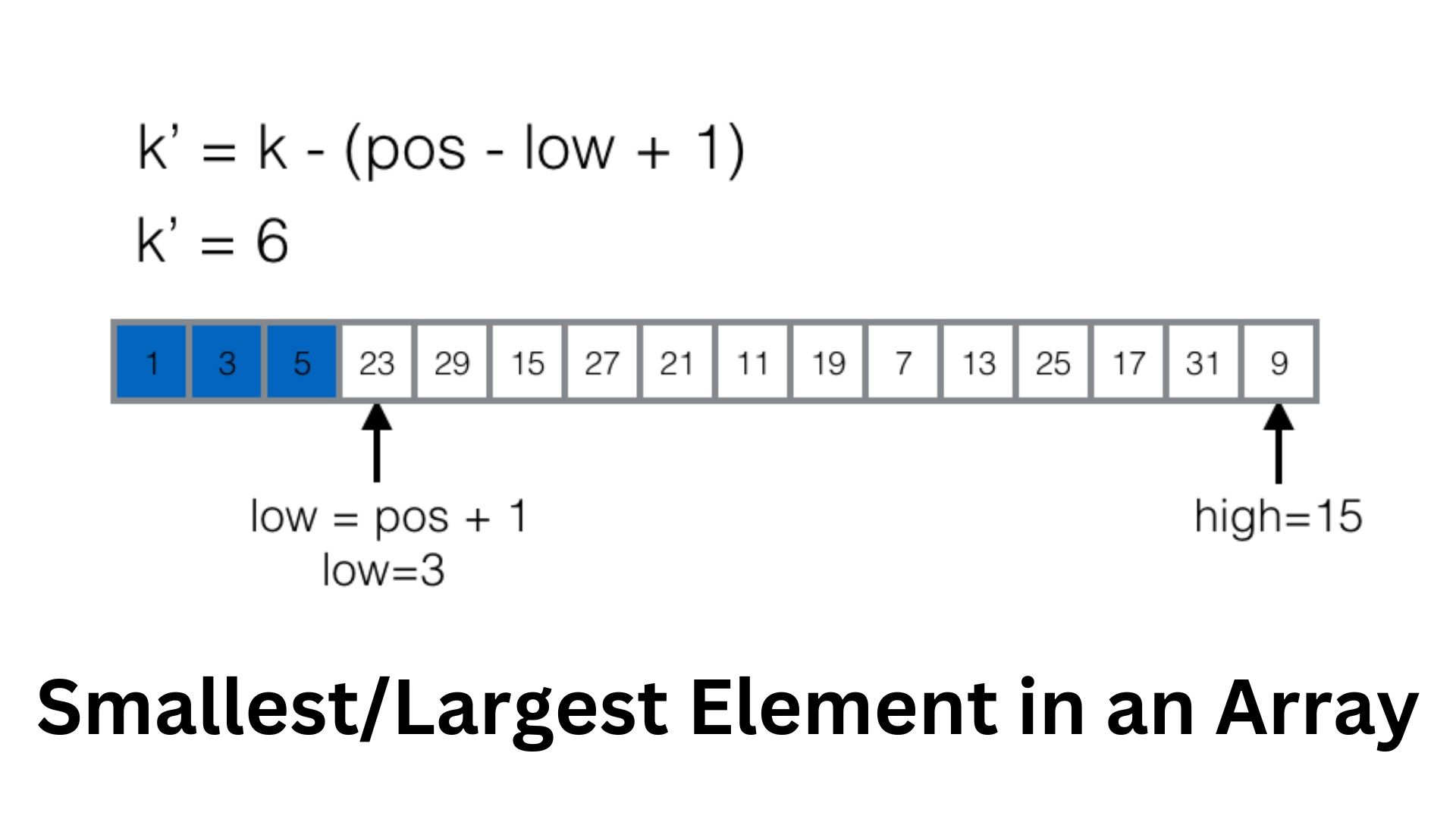# How to find the kth smallest/largest element in an array?## Overview

Given an unsorted array “arr”, find and print the “kth” smallest/largest element of the array.

Scope

• The method of sorting the array.
• The method of using a min-heap data structure.
• The method of using a max-heap data structure.

## By sorting the array

### Concept

We can sort the given array “arr”, and then access the element with index k. After sorting, for any valid index of arr “i”, the ith smallest element gets stored at the index i.

### Implementation

 #include "iostream"#include "vector"#include "algorithm"using namespace std;int findKthSmallest(vector&v, int k){    sort(begin(v), end(v));    return v[k - 1];}int main(){    vector arr = {10, 9, 1, 2, 5, 3, 4};    int k = 3;    cout << "The 3rd smallest element is : "<< findKthSmallest(arr, k)<
write your code here: Coding Playground

Output

 The 3rd smallest element is : 3

## Using Min-Heap

### Concept

Heap is also called a priority queue. It’s a data structure that stores elements in a sorted fashion. It provides access to only the top element in it viz the element with the highest priority. The order of the elements in a heap is determined by tier priority. But what is a priority?

It could be any parameter for the comparison of two data items of the same type. For example, the priority of two elements could be determined by checking which of them is larger, a string may have a higher priority over another if it is lexicographically smaller than the other, etc.

### Implementation

In this method, we shall use a min-heap. It always stores the elements in an order such that the smallest element is always available at the top. We push all the elements from the array in the min-heap. Then we remove the top element from the heap repeatedly k-1 times. Therefore the kth element that is present at the top of the heap is also the kth smallest element.

 #include "iostream"#include "vector"#include "queue"using namespace std;class cmp{    public:    bool operator()(const int&x, const int&y){        return x>y;    }};int findKthSmallest(vector&v, int k){    priority_queue, cmp>pq(begin(v), end(v));    while(--k>0){        pq.pop();    }    return pq.top();}int main(){    vector arr = {10, 9, 1, 2, 5, 3, 4};    int k = 3;    cout << "The 3rd smallest element is : "<< findKthSmallest(arr, k)<
write your code here: Coding Playground

Output

 The 3rd smallest element is : 3

## Using Max-Heap

### Concept

The concept here is similar to that in the above. The difference here is that instead of a min-heap, we use a max-heap. We iterate over the elements of the array, for each of which:
* We push it into the heap.
* If the size of the heap exceeds k, we pop off the top element of the heap.

At the end of this process, the smallest k elements of the given array will be present in the heap. The top of the heap is the kth smallest element as required, which we shall return.

### Implementation

 #include "iostream"#include "vector"#include "queue"using namespace std;int findKthSmallest(vector&v, int k){    priority_queuepq;    for(int it:v){        pq.push(it);        if(pq.size()>k){            pq.pop();        }    }    return pq.top();}int main(){    vector arr = {10, 9, 1, 2, 5, 3, 4};    int k = 3;    cout << "The 3rd smallest element is: "<< findKthSmallest(arr, k)<
write your code here: Coding Playground

Output

 The 3rd smallest element is : 3